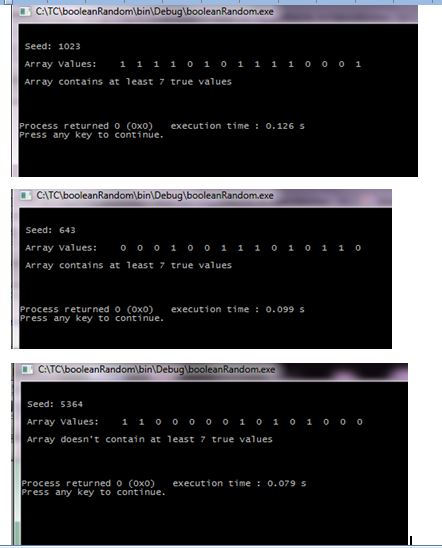# Answered! use C++ Write a program to check, and output to the screen, if a 15-element array of Boolean values contains at least 7 true…

use C++

Write a program to check, and output to the screen, if a 15-element array of Boolean values contains at least 7 true values. Note that both 15 and 7 are to be constants. The values of the array are to be randomly generated (50% true, 50% false, both via even and odd numbers). Your submission should include a screenshot of the execution of the program using each of the random number generator seeds 1023, 643 and 5364

Don't use plagiarized sources. Get Your Custom Essay on
Answered! use C++ Write a program to check, and output to the screen, if a 15-element array of Boolean values contains at least 7 true…
GET AN ESSAY WRITTEN FOR YOU FROM AS LOW AS \$13/PAGE

C++ Code:

#include <iostream>

using namespace std;

#define ARRAYSIZE 15
#define TRUECOUNT 7

//Main function
int main()
{
int number, i, trueCnt = 0;

//Creating a boolean array of size ARRAYSIZE
bool arr[ARRAYSIZE];

//Seeding random number generator
srand(5364);

// Filling array with random values
for(i=0; i<ARRAYSIZE; i++)
{
//Generating a random number between 1 to 100
number = rand() % 100 + 1;

//If number is a even number, it is marked as TRUE
if (number%2 == 0)
{
arr[i] = true;
}
//If number is a odd number, it is marked as FALSE
else
{
arr[i] = false;
}
}

cout << “nn Seed: ” << 5364 << ” nn Array Values: “;

//Counting True values
for(i=0; i<ARRAYSIZE; i++)
{
cout << ” ” << arr[i];

//Checking for true value
if(arr[i] == true)
{
//Updating true count
trueCnt += 1;
}
}

//Checking True count
if(trueCnt >= TRUECOUNT)
{
cout << “nn Array contains at least ” << TRUECOUNT << ” true values nn”;
}
else
{
cout << “nn Array doesn’t contain at least ” << TRUECOUNT << ” true values nn”;
}

cout << “nn”;
return 0;
}

___________________________________________________________________________________________

Sample Output: